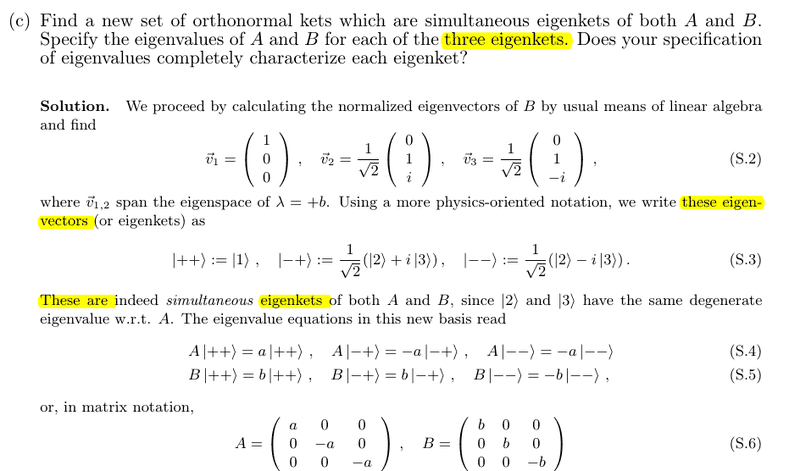# When should one eigenvector be split into two (same span)?

This question was inspired by 3c) on https://people.phys.ethz.ch/~muellrom/qm1_2012/Solutions4.pdf [Broken]

Given the operator
$$\hat{B} = \left(\matrix{b&0&0\\0&0&-ib\\0&ib&0}\right)$$

I find correctly that the eigenvalues are $\lambda = b, \pm b$.
To find the eigenvectors for $b$, I do the following

$$\left(\matrix{b&0&0\\0&0&-ib\\0&ib&0}\right) \left(\matrix{x\\y\\z}\right) = b \left(\matrix{x\\y\\z}\right)$$

$$bx = bx \hspace{10em} y = -iz \hspace{10em} z = iy\\$$
$$\hat{x} = \left(\matrix{t\\-iz\\iy}\right)\\ = \left(\matrix{t\\y\\iy}\right)$$

The pdf then seems to split this into two eigenvectors
$$\hat{x}_1 = \left(\matrix{t\\0\\0}\right) = \left(\matrix{1\\0\\0}\right) \hspace{5em} \text{and} \hspace{5em} \hat{x}_2 = \left(\matrix{0\\y\\iy}\right) = y\left(\matrix{0\\1\\i}\right)$$
which 'span the eigenspace' of $\lambda = b$.

Why is this allowed (separation of one eigenvector into multiple) and when should it be done?

Would it be technically acceptable to divide it further into $(1,0,0)$, $y(0,1,0)$ and $y(0,0,i)$? My current guess is that doing this would be acceptable but just not practical, and the reason the eigenvector here was divided into two was purely because of the $t$ making it difficult to factor the $y$ out. Is this right, or is there a deeper meaning I'm missing? (All these eigenvectors are pre-normalization)

Last edited by a moderator:

Simon Bridge
Homework Helper
You appear to have misunderstood what is going on.
The pdf author starts by finding the operator has 2 eigenvalues ... these are +b and -b.
The next step is to find out the conditions that x,y,z must satisfy if they are to be the components of an eigenvector that points to value +b.
The result is 3 simultanious equations that the author places, for convenience, into a vector form.
This vector is not an eigenvector.

The next step is to solve the equations to get the actual eigenvectors.
There are two vectors that satisfy the conditions: these are the sought-after eigenvectors.

The author has, at not time, split any eigenvectors up.

The result is 3 simultanious equations that the author places, for convenience, into a vector form.
This vector is not an eigenvector.
...
There are two vectors that satisfy the conditions: these are the sought-after eigenvectors..

Are you sure? The highlighted wording in the question makes me think otherwise.blue_leaf77
Homework Helper
Why is this allowed (separation of one eigenvector into multiple) and when should it be done?
It's about finding the basis for the null space of matrix
$$\left(\matrix{b&0&0\\0&0&-ib\\0&ib&0}\right) - \left(\matrix{b&0&0\\0&b&0\\0&0&b}\right) = \left(\matrix{0&0&0\\0&-b&-ib\\0&ib&-b}\right)$$
Using row reduction technique, you should find that any vector in the null space of the above matrix is
$$\left(\matrix{x\\y\\z}\right) = x\left(\matrix{1\\0\\0}\right) + y\left(\matrix{0\\1\\i}\right)$$
Which means the basis vectors are ##(1,0,0)^T## and ##(0,1,i)^T## and they also turn out to be already orthogonal.
Would it be technically acceptable to divide it further into $(1,0,0)$, $y(0,1,0)$ and $y(0,0,i)$?
You can't do that because the last two vectors are not in the null space of the above matrix.

•PerilousGourd
andrewkirk
Homework Helper
Gold Member
The solution
$$\hat{x} = \left(\matrix{t\\-iz\\iy}\right)= \left(\matrix{t\\y\\iy}\right)$$
contains two free variables: ##t## and ##y##. So any vector that can be written in that form, for any ##t,y\in\mathbb{C}##, is an eigenvector with eigenvalue ##b##. That tells us that the space of eigenvectors ('eigenspace') corresponding to eigenvalue ##b## is two-dimensional. To characterize that space we find a basis, which must have two elements. The obvious choice is the pair ## \vec v_1=\left(\matrix{1\\0\\0}\right),\ \vec v_2=\left(\matrix{0\\1\\i}\right)##, so that the solution above is equal to ##t\vec v_1+y\vec v_2##.
Would it be technically acceptable to divide it further into (1,0,0), y(0,1,0) and y(0,0,i)?
You can certainly write the solution as ##t\left(\matrix{1\\0\\0}\right)+y\left(\matrix{0\\1\\0}\right)+y\left(\matrix{0\\0\\i}\right)## but the second and third items are not in the eigenspace, so writing it in that way has no use. The aim is to write the solution as a sum of the basis elements of the eigenspace, and there can be only two basis elements because the eigenspace is two-dimensional.

•PerilousGourd
Simon Bridge
•